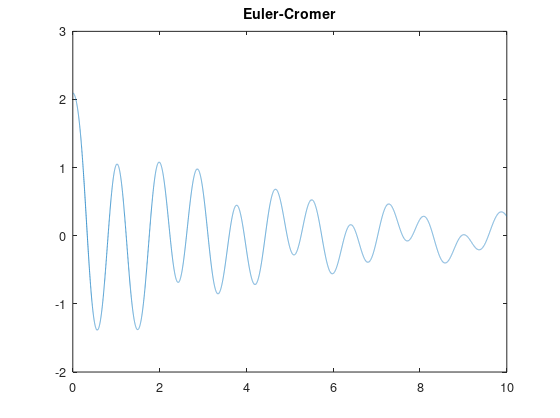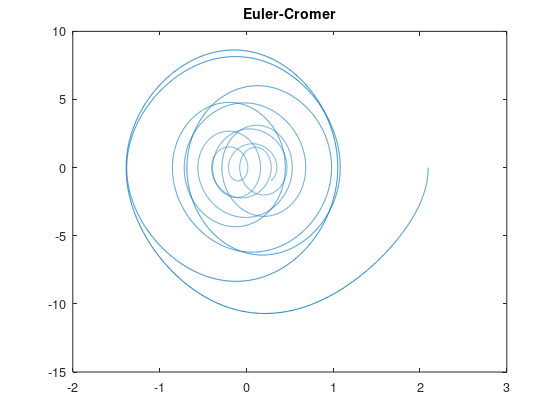# Hello! We are the Gavilan Engineering and Research (GEAR) Club

We are a welcoming space for people from any kind of background to come together and collaborate on projects they are passionate about. We are open for any Gavilan student to join, even if your major is not Engineering or STEM related.

## Latest Posts# Gavilan Aeronautics and Space Administration

Sept. 22, 2022, 8:24 p.m.

R-Candy

R-Candy is a sugar-based rocket fuel that combines sugar and Potassium Nitrate (KNO₃). We are researching a mixture that we think will be optimal for our rocket.

Rocket Body:

We are researching different ways to build our rocket body. Currently, we are exploring 3D printed options designed with CAD.Mk. 1 FuselageMk. 1 Nose Cone

Prototype 1# Buoyant Object Acceleration Physics

July 23, 2022, 12:13 a.m.

The main concept for this project is how buoyant objects behave in a fluid. Since the object is buoyant in the fluid (as given by the scenario) the fluid pushes the object from all sides, and it moves up. When the buoyant object and the fluid it is surrounded in accelerate, the fluid moves back because its container is accelerating and pushes it (Newton's 1st Law). Because the fluid is denser than the object, it moves back and pushes the object forward, creating an unusual effect. We hope to measure the change in the angle of the buoyant object and use that to find the acceleration.

The acceleration should = tan Ø, as given by this derivation:For information about the project's electronics go here

For information about the project's tracking software go here

For information about 3d modeling for the project go here# Euler Method Simulation - Enhanced Algorithm

June 14, 2022, 5:26 p.m.

June 17 2022

For the first part of this internship we compared the difference between Euler's method of integration with Euler-Cromer integration. It was observed that when using Euler's method in a Simple Harmonic Oscillator (SHO) the method would tend to increase the amount of energy in the system which is incorrect.The energy issue is solved by using the Euler-Cromer method.As shown on the figures, The Euler-Cromer method holds together much better and energy is conserved.

The code used is as follows.

 close all clear all clc % This script compares Euler's method of numerical integration to the analytical method. % Euler's method g = 9.81; %acceleration of gravity [m/s^2]. m = 1; %mass of the object [kg]. k = 1; %spring constant [N/m]. y0 = 5; %initial position in relation to equilibrium [m]. v0 = 0; %initial velocity [m/s]. dt = 0.01; %time interval between steps [s]. n = 10000; %number of steps. t(1) = 0; %initial time condition. y(1) = y0; %initial position condition. v(1) = v0; %initial velocity condition. pe(1) = .5 * k * y0^2; ke(1) = .5 * m * v0^2; e(1) = pe(1) + ke(1); for i = 2:n   t(i) = t(i-1) + dt; % adds time interval to previous time and saves the value in a vector.   v(i) = v(i-1) - (k./m) .* y(i-1) .* dt; %Calculates new velocity and saves the value in a vector.   y(i) = y(i-1) + v(i-1) * dt; %Calculates new position and saves the value in a vector.   pe(i) = .5 * k * y(i)^2;   ke(i) = .5 * m * v(i)^2;   e(i) = pe(i) + ke(i); end figure 1 plot(t,v,'r',t,y,'b') title("Euler's method of numerical integration") legend('Velocity [m/s]','position [m]') %----------------------------------------% % Analytical method v_exact = -y0 * sqrt(k ./ m) .* sin(sqrt(k ./ m) .* t); y_exact = y0 * cos(sqrt(k ./ m) .* t); pe_exact = .5 .* k .* y_exact .^ 2; ke_exact = .5 .* m .* v_exact .^ 2; e_exact = pe_exact + ke_exact; figure 2  plot(t,v_exact,'r',t,y_exact,'b',t,v,'r--',t,y,'b--',t,e,'g',t,e_exact,'y') title('Euler vs exact') legend('v exact','y exact','v Euler','y Euler','Energy Euler','Exact Energy') close all clear all clc % This script compares the Euler-cromer method of numerical integration to the analytical method. % Euler-cromer method g = 9.81; %acceleration of gravity [m/s^2]. m = 1; %mass of the object [kg]. k = 1; %spring constant [N/m]. y0 = 5; %initial position in relation to equilibrium [m]. v0 = 0; %initial velocity [m/s]. dt = 0.01; %time interval between steps [s]. n = 10000; %number of steps. t(1) = 0; %initial time condition. y(1) = y0; %initial position condition. v(1) = v0; %initial velocity condition. pe(1) = .5 * k * y0^2; %initial spring potential energy. ke(1) = .5 * m * v0^2; %initial object kinetic energy. e(1) = pe(1) + ke(1); %initial total energy. F(1) = -k * y0; %initial force on the object. for i = 2:n   t(i) = t(i-1) + dt; % adds time interval to previous time and saves the value in a vector.   F(i) = -k .* y(i-1); % calculates the force on the object.   a(i) = F(i) / m; % calculates the accelerationon of the object.   v(i) = v(i-1) + a(i) * dt; % calculatesthe velocity of the object.   y(i) = y(i-1) + v(i) * dt; %calculates the position of the object.   pe(i) = .5 * k * y(i)^2; % calculates object potential energy.   ke(i) = .5 * m * v(i)^2; % calculates object kinetic energy.   e(i) = pe(i) + ke(i); %calculates object total energy. end %----------------------------------------% % Analytical method v_exact = -y0 * sqrt(k ./ m) .* sin(sqrt(k ./ m) .* t); y_exact = y0 * cos(sqrt(k ./ m) .* t); pe_exact = .5 .* k .* y_exact .^ 2; ke_exact = .5 .* m .* v_exact .^ 2; e_exact = pe_exact + ke_exact; plot(t,y_exact,'b',t,y,'r--',t,e,'g',t,e_exact,'y') title('Euler vs exact') legend('y exact','y Euler','Energy Euler','Exact Energy')

June 24 2022

During this week we analyzed the Euler-Cromer method of numeric integration in a rigid pendulum. We also added resistance and oscillatory forces into our system.As you can see the graphs are a lot more chaotic.

The code used is as follows:

 clc clear all close all g = 9.81; L = 2; m = 5; b = 3; I = (m * L^2) / 3; Tau = 10; w = 2.5; omega(1) = 0; theta(1) = 120 * pi / 180; alpha(1) =  ((3 * g) / (2 * L))^2 * sin(theta(1)); nsteps = 10000; dt = .001; t(1) = 0; for n = 2:nsteps    t(n) = t(n-1) + dt;   alpha(n) = -((3 * g) / (2 * L))^2 * sin(theta(n-1)) - (b*omega(n-1))/I + Tau * cos(w*t(n));   omega(n) = omega(n-1) + alpha(n) * dt;   theta(n) = theta(n-1) + omega(n) * dt; end plot(t,theta) title('Euler-Cromer') figure 2 plot(theta, omega) title('Euler-Cromer')

July 1 2022

This week we studied the relationship between a cubic oscillator’s period and its amplitude. We calculated that the period of the oscillator is proportional to the inverse of the amplitude.We also worked with animation and modeling techniques in Matlab.

This code calculates and animates a rigid pendulum.

 clc clear all close all %%% This code models and animates the motion of a rigid pendulum. g = 9.81; %gravity L = 2; % length m = 5; % mass b = 0; % resistance I = (m * L^2) / 3; %moment of inirtia Tau = 0; % added force w = 2.5; % added force interval omega(1) = 0; %initialize omega (initial velocity) theta(1) = 30 * pi / 180; % initialize theta (initial angle) alpha(1) = ((3 * g) / (2 * L))^2 * sin(theta(1)); %initialize alpha (initial acceleration) nsteps = 1000; % sets number of steps dt = .01; % sets time step t(1) = 0; %initialize time % Euler-Cromer method for n = 2:nsteps t(n) = t(n-1) + dt; alpha(n) = -((3 * g) / (2 * L))^2 * sin(theta(n-1)) - (b*omega(n-1))/I + Tau * cos(w*t(n)); omega(n) = omega(n-1) + alpha(n) * dt; theta(n) = theta(n-1) + omega(n) * dt; end plot(t,theta) title('Euler-Cromer') figure plot(theta, omega) title('Euler-Cromer') X = -.5 * L .* sin(theta); % locates center of mass in the x direction Y = -.5 * L .* cos(theta); % locates center of mass in the y direction figure % animation for the pendulum for z = 1:length(theta) plot ([0,2*X(z)],[0,2*Y(z)],'linewidth',2) axis([-2,2,-2,0]) hold on plot(X(z),Y(z),'ro') pause(.01) %storing frames K(z) = getframe(gcf); hold off end videofile = VideoWriter('pendulum.avi','Uncompressed AVI') open(videofile) writeVideo(videofile,K) close(videofile)

I am also working on a simulation of the ISS orbiting Earth, but I have run into an issue when calculating the angle and I have not been able to figure it out yet. Here is my code so far. This is still very rough.

 clc close all clear all mass_Earth = 5.972e24; mass_object = 20000; x(1) = 0; y(1) = 6786100; alt(1) = sqrt(x(1)^2 + y(1)^2); vx(1) = 7778; vy(1) = 0; ax(1) = 0; ay(1) = 0; nsteps = 100; dt = 60; t(1) = 0; theta(1) = 0; for n = 2:nsteps f(n) = -6.67428e-11 * (mass_Earth * mass_object) / alt(n-1)^2; fx(n) = f(n) * sin(theta(n-1)); fy(n) = f(n) * cos(theta(n-1)); ax(n) = fx(n)/mass_object; ay(n) = fy(n)/mass_object; vx(n) = vx(n-1) + ax(n) * dt; vy(n) = vy(n-1) + ay(n) * dt; x(n) = x(n-1) + vx(n) * dt; y(n) = y(n-1) + vy(n) * dt; alt(n) = sqrt(x(n)^2 + y(n)^2); v(n) = sqrt(vx(n)^2 + vy(n)^2); theta(n) = theta(n-1) + (v(n)/(2 * pi * alt(n)))*dt; plot(x(n),y(n),'rx','LineWidth',10) axis([-7000000,7000000,-7000000,7000000]) pause(.1) t(n) = t(n-1)+dt; end

July 15 2022

This week we worked on waves as a function of displacement and time. We were able to come up with a function to predict the future state of a wave.

future(n) = r2(current(n+1)+current(n-1))+2(1-r2)current(n)-past(n)

We applied this function to many different initial conditions including: fixed point “plucked” string, oscillating ends, and “sliding” end string.